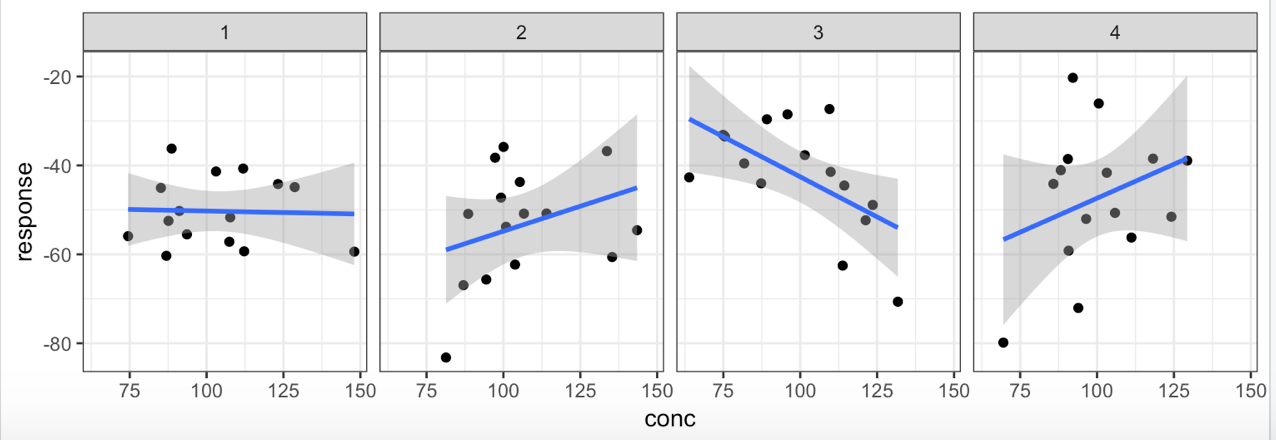# An article in a statistics or medical journal, “Using Simulations to Convince People of the Importance of Random Variation When Interpreting Statistics.”Andy Stein writes:

On one of my projects, I had a plot like the one above of drug concentration vs response, where we divided the patients into 4 groups. I look at the data below and think “wow, these are some wide confidence intervals and random looking data, let’s not spend too much time more with this” but many others do not agree and we spend lots of time on plots like this. Next time, I’ll do what I finally did today and simulate random noise and show how trends pop up. But I was just wondering if you had any advice on how to teach scientists about how easy it is to find trends in randomness? I’m thinking of writing a little shiny app that lets you tune some of the parameters in the R code below to create different versions of this plot. But I know I can’t be the first person to have this challenge and I thought maybe you or others have given some thought on how to teach this concept – that one can easily find trends in randomly generated data if the sample size is small enough and if you create enough plots.

This all makes sense to me. An ideal outcome would be for Stein and others to write an article for a journal such as Technometrics or American Statistician with a title such as “Using Simulations to Convince People of the Importance of Random Variation When Interpreting Statistics.”

Stein replied:

My ideal outcome would be for there to be an article with luminaries in the field in a journal like NEJM or Lancet where everyone will have to pay attention. Unfortunately, I don’t think my colleagues give enough weight to what’s in the statistics and pharmacometrics journals. If they did, then I wouldn’t be in this predicament! Having such a paper out in the world in a high-impact journal would be enough to make me really happy.

My latest idea is to make a little Shiny App where you upload your dataset and it creates 10 sets of plots – one of the real data and nine where the Y variable has been randomly permuted across all the groups. Then people get to see if they can pick out the real data from the mix. If I get a public version implemented in time, I’ll try and send that along, too.

Here’s Stein’s R code:

```set.seed(1)
n_subjects = 15
n_trials   = 4

mean_response = -50
sd_response   = 15
mean_conc = 100
sd_conc   = 20

rand_values = data.frame(response = rnorm(n = n_subjects*n_trials, mean = mean_response, sd = sd_response),
conc     = rnorm(n = n_subjects*n_trials, mean = mean_conc, sd = sd_conc),
id       = rep(1:n_trials,times = n_subjects))
g = ggplot(data = rand_values, aes(x=conc,y=response))
g = g + geom_point()
g = g + geom_smooth(method = "lm")
g = g + facet_wrap(~id)
print(g)
```